# It is known that a force with a moment of 960 N · m about D is required to straighten the fence post CD. If d = 2.80 m, determine the tension that must be developed in the cable of winch puller AB to create the required moment about Point D. Please give a detailed explanation

Question-AnswerCategory: Engineering MechanicsIt is known that a force with a moment of 960 N · m about D is required to straighten the fence post CD. If d = 2.80 m, determine the tension that must be developed in the cable of winch puller AB to create the required moment about Point D. Please give a detailed explanation

It is known that a force with a moment of 960 N · m about D is required to straighten the fence post CD. If d = 2.80 m, determine the tension that must be developed in the cable of winch puller AB to create the required moment about Point D. Please give a detailed explanationStep: 1

Draw the free body diagram of the fence post as follows: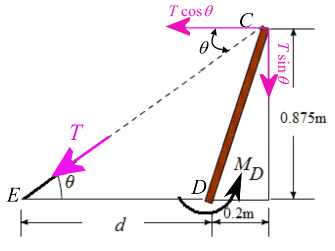Step: 2

Let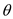be the angle exerted by the cable with the horizontal.
Determine the angle as follows: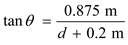Substitute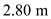for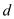.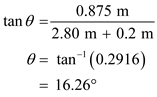Step: 3

Let the horizontal component of the tension in the cable BC be.
Resolve the tension in the cable as below and obtain the horizontal component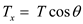Substitute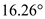for.Step: 4

Resolve the tension in the cable and obtain the vertical component of the tension,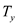in the cable BC.Substitutefor.Step: 5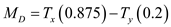Here,is the moment needed for the fence post to straighten.
Substitutefor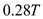forand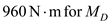.Therefore, the tension in the cable BC is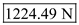.